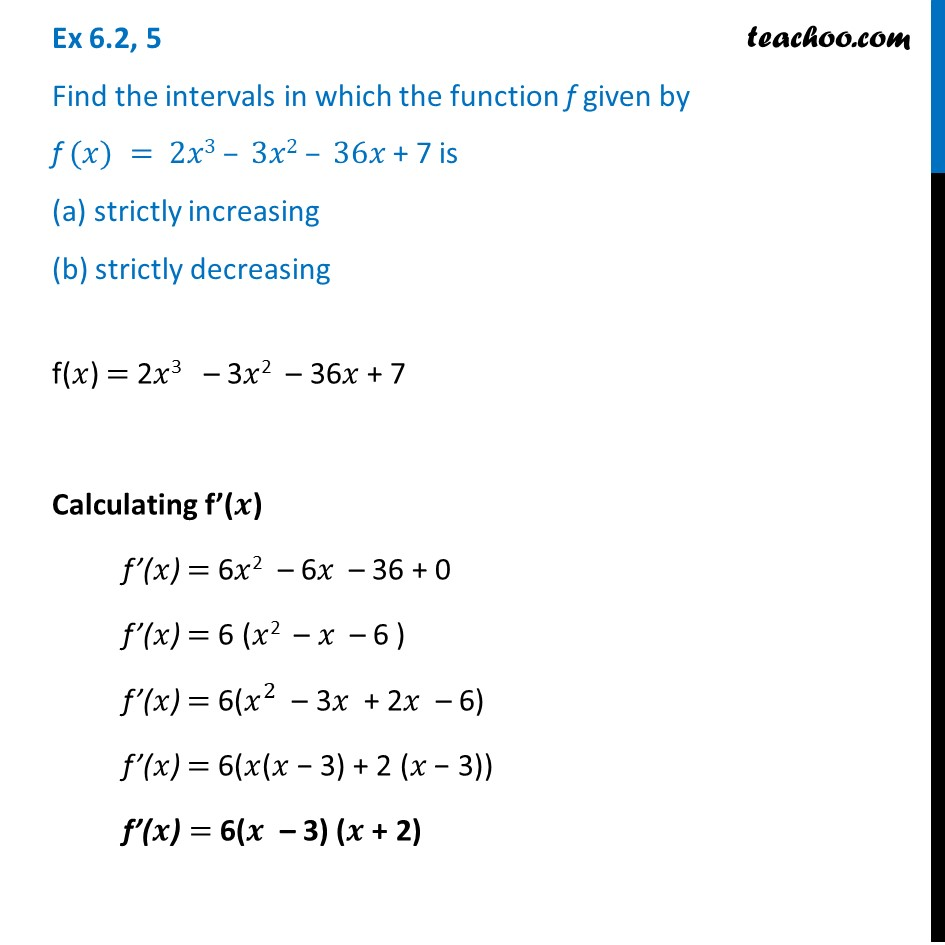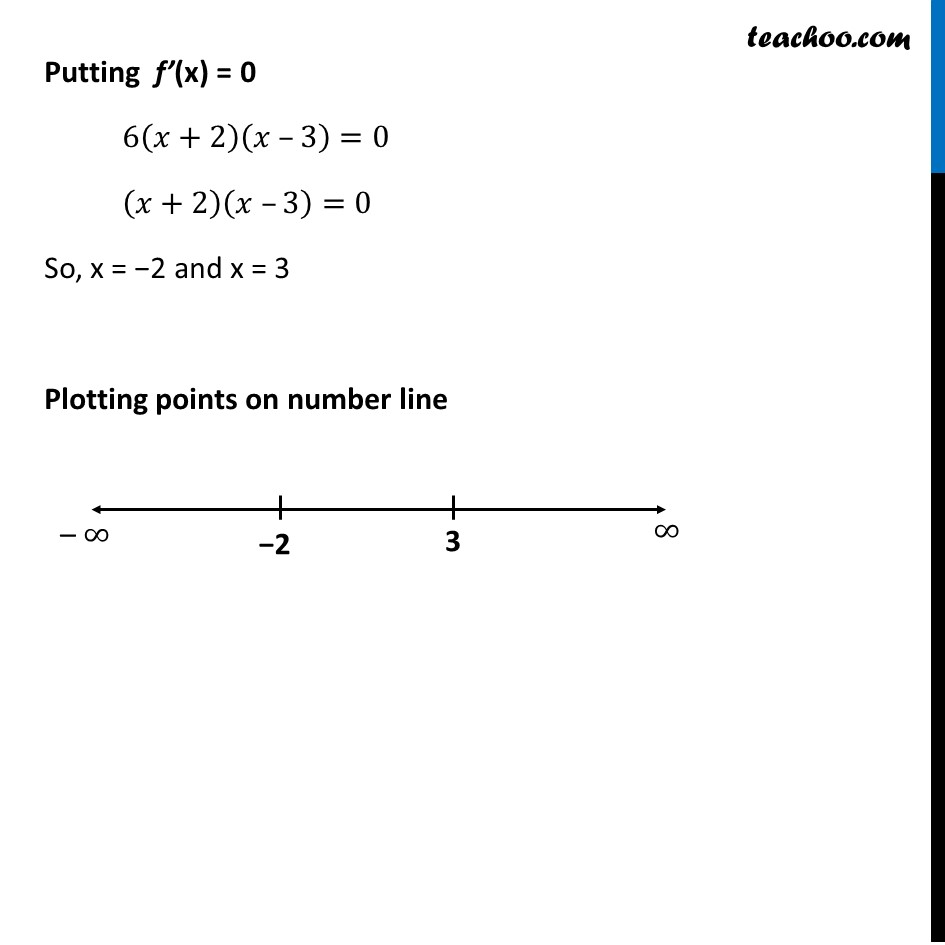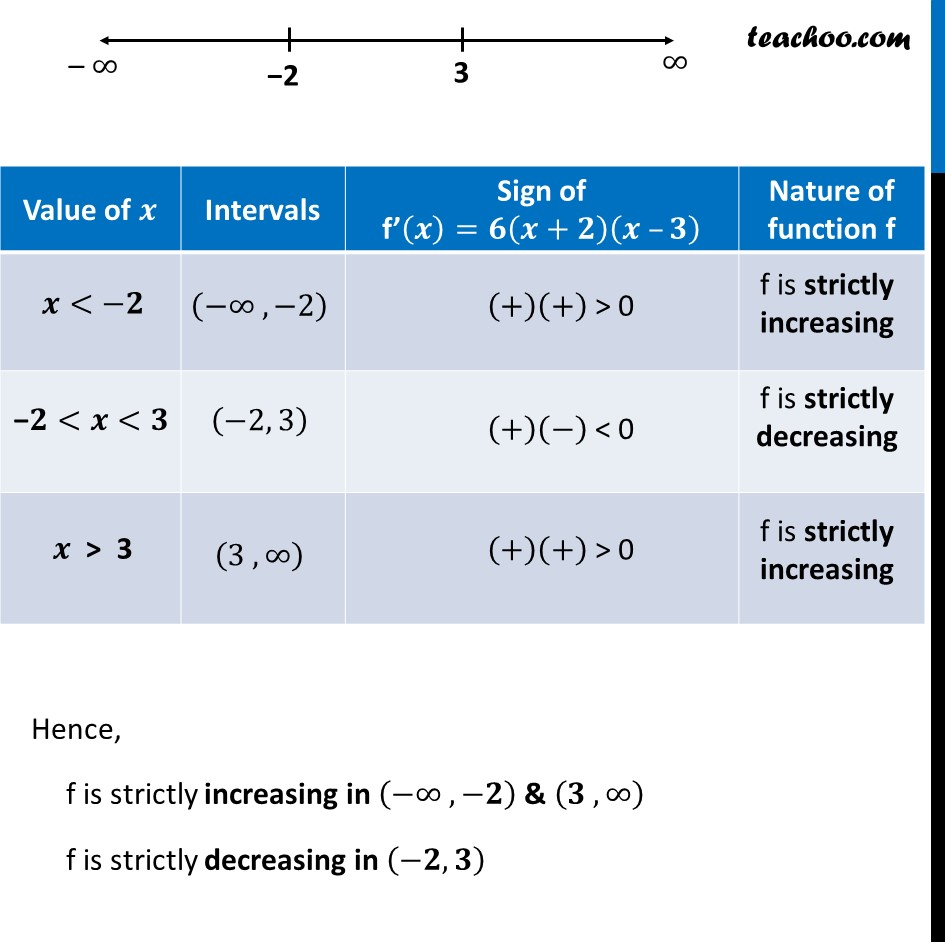Ex 6.2

Chapter 6 Class 12 Application of Derivatives
Serial order wiseLearn in your speed, with individual attention - Teachoo Maths 1-on-1 Class

### Transcript

Ex 6.2, 5 Find the intervals in which the function f given by f (𝑥) = 2𝑥3 – 3𝑥2 – 36𝑥 + 7 is (a) strictly increasing (b) strictly decreasingf(𝑥) = 2𝑥3 – 3𝑥2 – 36𝑥 + 7 Calculating f’(𝒙) f’(𝑥) = 6𝑥2 – 6𝑥 – 36 + 0 f’(𝑥) = 6 (𝑥2 – 𝑥 – 6 ) f’(𝑥) = 6(𝑥^2 – 3𝑥 + 2𝑥 – 6) f’(𝑥) = 6(𝑥(𝑥 − 3) + 2 (𝑥 − 3)) f’(𝒙) = 6(𝒙 – 3) (𝒙 + 2) Putting f’(x) = 0 6(𝑥+2)(𝑥 –3)=0 (𝑥+2)(𝑥 –3)=0 So, x = −2 and x = 3 Plotting points on number line Hence, f is strictly increasing in (−∞ ,−𝟐) & (𝟑 ,∞) f is strictly decreasing in (−𝟐, 𝟑)